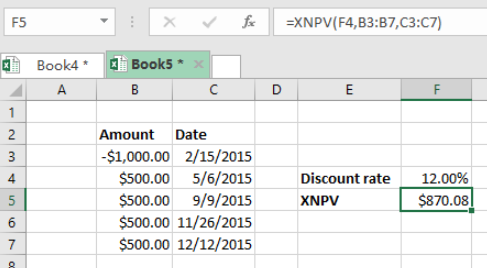Get instant live expert help with Excel or Google Sheets“My Excelchat expert helped me in less than 20 minutes, saving me what would have been 5 hours of work!”

#### Post your problem and you’ll get expert help in seconds.

Your message must be at least 40 characters
Our professional experts are available now. Your privacy is guaranteed.

# Pro Tips on Using the XNPV Function in Excel

We need the Excel XNPV function to calculate the net present value of a given investment with a given discount rate when the cash flows come at an irregular intervals. Here are pro tips on using the Excel XNPV function in excel to get the NPV given irregular cash flowsFigure 1: How to use XNPV to find NPV with irregular cash flows

## General syntax of the formula

`=XNPV (rate, values, dates)`

Where;

• Rate- this is the discount rate to be applied on the cash flow
• Value – values that represent the cash flows
• Dates- fates corresponding to cash flows.

## Understanding the formula

• The above formula can help us calculate the NPV for an investment when the cash flows are irregular given a certain interest rate.
• To work with the function, we need to first arrange the dates in a chronological order.
• The negative cash flows will represent the cash paid out, while the positive value represent cash received.
• In our example above, we have our formula in cell F5, which is `=XNPV(F4,B3:B7,C3:C7)`
• It is important to note that the XNPV does not discount the initial cash flow.
• All the other subsequent payments are discounted based on a 365-day year.
• If you want to discount a given valuation date, you should set up the XNPV so that the first cash flow which is associated with the valuation date is zero.
• It is also necessary to note that the XNPV function can only work correctly when valid excel dates are used, and should be arranged in a chronological order.

## Instant Connection to an Expert through our Excelchat Service

Most of the time, the problem you will need to solve will be more complex than a simple application of a formula or function. If you want to save hours of research and frustration, try our live Excelchat service! Our Excel Experts are available 24/7 to answer any Excel question you may have. We guarantee a connection within 30 seconds and a customized solution within 20 minutes.

Solution examplesI have a figure which is the sum of 9 months of payments. Each payment decreases by the same amount (X) so the first payment is Y, second payment is Y-X, third is Y-(Xx2), forth is Y-(Xx3) and so on. I am trying to calculate what each of the 9 payments should be.
Solved by A. W. in 60 mins